Computes a single, double or triple integral over a given 1D interval, 2D rectangle, or 3D cuboid. A basic example:

>>> from mpmath import *
>>> mp.dps = 15; mp.pretty = True
2.0


A basic 2D integral:

>>> f = lambda x, y: cos(x+y/2)
>>> quad(f, [-pi/2, pi/2], [0, pi])
4.0


Interval format

The integration range for each dimension may be specified using a list or tuple. Arguments are interpreted as follows:

quad(f, [x1, x2]) – calculates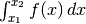quad(f, [x1, x2], [y1, y2]) – calculates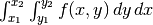quad(f, [x1, x2], [y1, y2], [z1, z2]) – calculates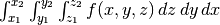Endpoints may be finite or infinite. An interval descriptor may also contain more than two points. In this case, the integration is split into subintervals, between each pair of consecutive points. This is useful for dealing with mid-interval discontinuities, or integrating over large intervals where the function is irregular or oscillates.

Options

quad() recognizes the following keyword arguments:

method
Chooses integration algorithm (described below).
error
If set to true, quad() returns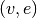where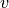is the integral and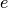is the estimated error.
maxdegree
Maximum degree of the quadrature rule to try before quitting.
verbose

Algorithms

Mpmath presently implements two integration algorithms: tanh-sinh quadrature and Gauss-Legendre quadrature. These can be selected using method=’tanh-sinh’ or method=’gauss-legendre’ or by passing the classes method=TanhSinh, method=GaussLegendre. The functions quadts() and quadgl() are also available as shortcuts.

Both algorithms have the property that doubling the number of evaluation points roughly doubles the accuracy, so both are ideal for high precision quadrature (hundreds or thousands of digits).

At high precision, computing the nodes and weights for the integration can be expensive (more expensive than computing the function values). To make repeated integrations fast, nodes are automatically cached.

The advantages of the tanh-sinh algorithm are that it tends to handle endpoint singularities well, and that the nodes are cheap to compute on the first run. For these reasons, it is used by quad() as the default algorithm.

Gauss-Legendre quadrature often requires fewer function evaluations, and is therefore often faster for repeated use, but the algorithm does not handle endpoint singularities as well and the nodes are more expensive to compute. Gauss-Legendre quadrature can be a better choice if the integrand is smooth and repeated integrations are required (e.g. for multiple integrals).

See the documentation for TanhSinh and GaussLegendre for additional details.

Examples of 1D integrals

Intervals may be infinite or half-infinite. The following two examples evaluate the limits of the inverse tangent function (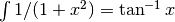), and the Gaussian integral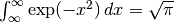:

>>> mp.dps = 15
>>> quad(lambda x: 2/(x**2+1), [0, inf])
3.14159265358979
>>> quad(lambda x: exp(-x**2), [-inf, inf])**2
3.14159265358979


Integrals can typically be resolved to high precision. The following computes 50 digits of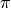by integrating the area of the half-circle defined by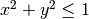,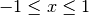,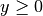:

>>> mp.dps = 50
>>> 2*quad(lambda x: sqrt(1-x**2), [-1, 1])
3.1415926535897932384626433832795028841971693993751


One can just as well compute 1000 digits (output truncated):

>>> mp.dps = 1000
>>> 2*quad(lambda x: sqrt(1-x**2), [-1, 1])
3.141592653589793238462643383279502884...216420198


Complex integrals are supported. The following computes a residue at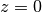by integrating counterclockwise along the diamond-shaped path fromto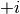to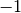to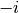to:

>>> mp.dps = 15
(0.0 + 6.28318530717959j)


Examples of 2D and 3D integrals

Here are several nice examples of analytically solvable 2D integrals (taken from MathWorld ) that can be evaluated to high precision fairly rapidly by quad():

>>> mp.dps = 30
>>> f = lambda x, y: (x-1)/((1-x*y)*log(x*y))
>>> quad(f, [0, 1], [0, 1])
0.577215664901532860606512090082
>>> +euler
0.577215664901532860606512090082

>>> f = lambda x, y: 1/sqrt(1+x**2+y**2)
>>> quad(f, [-1, 1], [-1, 1])
3.17343648530607134219175646705
>>> 4*log(2+sqrt(3))-2*pi/3
3.17343648530607134219175646705

>>> f = lambda x, y: 1/(1-x**2 * y**2)
>>> quad(f, [0, 1], [0, 1])
1.23370055013616982735431137498
>>> pi**2 / 8
1.23370055013616982735431137498

>>> quad(lambda x, y: 1/(1-x*y), [0, 1], [0, 1])
1.64493406684822643647241516665
>>> pi**2 / 6
1.64493406684822643647241516665


Multiple integrals may be done over infinite ranges:

>>> mp.dps = 15
>>> print(quad(lambda x,y: exp(-x-y), [0, inf], [1, inf]))
0.367879441171442
>>> print(1/e)
0.367879441171442


For nonrectangular areas, one can call quad() recursively. For example, we can replicate the earlier example of calculatingby integrating over the unit-circle, and actually use double quadrature to actually measure the area circle:

>>> f = lambda x: quad(lambda y: 1, [-sqrt(1-x**2), sqrt(1-x**2)])
3.14159265358979


Here is a simple triple integral:

>>> mp.dps = 15
>>> f = lambda x,y,z: x*y/(1+z)
>>> quad(f, [0,1], [0,1], [1,2], method='gauss-legendre')
0.101366277027041
>>> (log(3)-log(2))/4
0.101366277027041


Singularities

Both tanh-sinh and Gauss-Legendre quadrature are designed to integrate smooth (infinitely differentiable) functions. Neither algorithm copes well with mid-interval singularities (such as mid-interval discontinuities in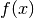or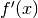). The best solution is to split the integral into parts:

>>> mp.dps = 15
3.99900894176779
>>> quad(lambda x: abs(sin(x)), [0, pi, 2*pi])  # Good
4.0


The tanh-sinh rule often works well for integrands having a singularity at one or both endpoints:

>>> mp.dps = 15
>>> quad(log, [0, 1], method='tanh-sinh')  # Good
-1.0
-0.999932197413801


However, the result may still be inaccurate for some functions:

>>> quad(lambda x: 1/sqrt(x), [0, 1], method='tanh-sinh')
1.99999999946942


This problem is not due to the quadrature rule per se, but to numerical amplification of errors in the nodes. The problem can be circumvented by temporarily increasing the precision:

>>> mp.dps = 30
>>> a = quad(lambda x: 1/sqrt(x), [0, 1], method='tanh-sinh')
>>> mp.dps = 15
>>> +a
2.0


Highly variable functions

For functions that are smooth (in the sense of being infinitely differentiable) but contain sharp mid-interval peaks or many “bumps”, quad() may fail to provide full accuracy. For example, with default settings, quad() is able to integrateaccurately over an interval of length 100 but not over length 1000:

>>> quad(sin, [0, 100]); 1-cos(100)   # Good
0.137681127712316
0.137681127712316
-37.8587612408485
0.437620923709297


One solution is to break the integration into 10 intervals of length 100:

>>> quad(sin, linspace(0, 1000, 10))   # Good
0.437620923709297


Another is to increase the degree of the quadrature:

>>> quad(sin, [0, 1000], maxdegree=10)   # Also good
0.437620923709297


Whether splitting the interval or increasing the degree is more efficient differs from case to case. Another example is the function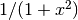, which has a sharp peak centered around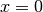:

>>> f = lambda x: 1/(1+x**2)
3.64804647105268
>>> quad(f, [-100, 100], maxdegree=10)   # Good
3.12159332021646
>>> quad(f, [-100, 0, 100])   # Also good
3.12159332021646


References

mpmath.quadosc(ctx, f, interval, omega=None, period=None, zeros=None)

Calculates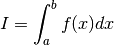where at least one of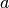and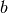is infinite and where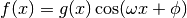for some slowly decreasing function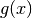. With proper input, quadosc() can also handle oscillatory integrals where the oscillation rate is different from a pure sine or cosine wave.

In the standard case when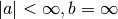, quadosc() works by evaluating the infinite series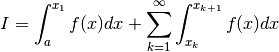where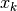are consecutive zeros (alternatively some other periodic reference point) of. Accordingly, quadosc() requires information about the zeros of. For a periodic function, you can specify the zeros by either providing the angular frequency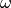(omega) or the period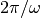. In general, you can specify the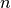-th zero by providing the zeros arguments. Below is an example of each:

>>> from mpmath import *
>>> mp.dps = 15; mp.pretty = True
>>> f = lambda x: sin(3*x)/(x**2+1)
0.37833007080198
0.37833007080198
>>> quadosc(f, [0,inf], zeros=lambda n: pi*n/3)
0.37833007080198
>>> (ei(3)*exp(-3)-exp(3)*ei(-3))/2  # Computed by Mathematica
0.37833007080198


Note that zeros was specified to multiplyby the half-period, not the full period. In theory, it does not matter whether each partial integral is done over a half period or a full period. However, if done over half-periods, the infinite series passed to nsum() becomes an alternating series and this typically makes the extrapolation much more efficient.

Here is an example of an integration over the entire real line, and a half-infinite integration starting at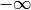:

>>> quadosc(lambda x: cos(x)/(1+x**2), [-inf, inf], omega=1)
1.15572734979092
>>> pi/e
1.15572734979092
>>> quadosc(lambda x: cos(x)/x**2, [-inf, -1], period=2*pi)
-0.0844109505595739
>>> cos(1)+si(1)-pi/2
-0.0844109505595738


Of course, the integrand may contain a complex exponential just as well as a real sine or cosine:

>>> quadosc(lambda x: exp(3*j*x)/(1+x**2), [-inf,inf], omega=3)
(0.156410688228254 + 0.0j)
>>> pi/e**3
0.156410688228254
>>> quadosc(lambda x: exp(3*j*x)/(2+x+x**2), [-inf,inf], omega=3)
(0.00317486988463794 - 0.0447701735209082j)
>>> 2*pi/sqrt(7)/exp(3*(j+sqrt(7))/2)
(0.00317486988463794 - 0.0447701735209082j)


Non-periodic functions

If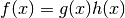for some function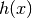that is not strictly periodic, omega or period might not work, and it might be necessary to use zeros.

A notable exception can be made for Bessel functions which, though not periodic, are “asymptotically periodic” in a sufficiently strong sense that the sum extrapolation will work out:

>>> quadosc(j0, [0, inf], period=2*pi)
1.0
1.0


More properly, one should provide the exact Bessel function zeros:

>>> j0zero = lambda n: findroot(j0, pi*(n-0.25))
1.0


For an example where zeros becomes necessary, consider the complete Fresnel integrals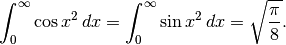Although the integrands do not decrease in magnitude as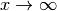, the integrals are convergent since the oscillation rate increases (causing consecutive periods to asymptotically cancel out). These integrals are virtually impossible to calculate to any kind of accuracy using standard quadrature rules. However, if one provides the correct asymptotic distribution of zeros (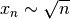), quadosc() works:

>>> mp.dps = 30
>>> f = lambda x: cos(x**2)
0.626657068657750125603941321203
>>> f = lambda x: sin(x**2)
0.626657068657750125603941321203
>>> sqrt(pi/8)
0.626657068657750125603941321203


(Interestingly, these integrals can still be evaluated if one places some other constant thanin the square root sign.)

In general, if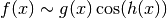, the zeros follow the inverse-function distribution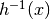:

>>> mp.dps = 15
>>> f = lambda x: sin(exp(x))
>>> quadosc(f, [1,inf], zeros=lambda n: log(n))
-0.25024394235267
>>> pi/2-si(e)
-0.250243942352671


Non-alternating functions

If the integrand oscillates around a positive value, without alternating signs, the extrapolation might fail. A simple trick that sometimes works is to multiply or divide the frequency by 2:

>>> f = lambda x: 1/x**2+sin(x)/x**4
1.28642190869861
>>> quadosc(f, [1,inf], omega=0.5)  # Perfect
1.28652953559617
>>> 1+(cos(1)+ci(1)+sin(1))/6
1.28652953559617


Fast decay

quadosc() is primarily useful for slowly decaying integrands. If the integrand decreases exponentially or faster, quad() will likely handle it without trouble (and generally be much faster than quadosc()):

>>> quadosc(lambda x: cos(x)/exp(x), [0, inf], omega=1)
0.5
>>> quad(lambda x: cos(x)/exp(x), [0, inf])
0.5


Quadrature rules are implemented using this class, in order to simplify the code and provide a common infrastructure for tasks such as error estimation and node caching.

You can implement a custom quadrature rule by subclassing QuadratureRule and implementing the appropriate methods. The subclass can then be used by quad() by passing it as the method argument.

QuadratureRule instances are supposed to be singletons. QuadratureRule therefore implements instance caching in __new__().

calc_nodes(degree, prec, verbose=False)

Compute nodes for the standard interval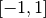. Subclasses should probably implement only this method, and use get_nodes() method to retrieve the nodes.

clear()

Delete cached node data.

estimate_error(results, prec, epsilon)

Given results from integrations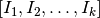done with a quadrature of rule of degree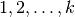, estimate the error of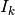.

For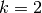, we estimate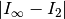as.

For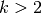, we extrapolate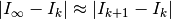from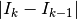and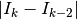under the assumption that each degree increment roughly doubles the accuracy of the quadrature rule (this is true for both TanhSinh and GaussLegendre). The extrapolation formula is given by Borwein, Bailey & Girgensohn. Although not very conservative, this method seems to be very robust in practice.

get_nodes(a, b, degree, prec, verbose=False)

Return nodes for given interval, degree and precision. The nodes are retrieved from a cache if already computed; otherwise they are computed by calling calc_nodes() and are then cached.

Subclasses should probably not implement this method, but just implement calc_nodes() for the actual node computation.

guess_degree(prec)

Given a desired precision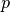in bits, estimate the degree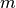of the quadrature required to accomplish full accuracy for typical integrals. By default, quad() will perform up toiterations. The value ofshould be a slight overestimate, so that “slightly bad” integrals can be dealt with automatically using a few extra iterations. On the other hand, it should not be too big, so quad() can quit within a reasonable amount of time when it is given an “unsolvable” integral.

The default formula used by guess_degree() is tuned for both TanhSinh and GaussLegendre. The output is roughly as follows:50 6
100 7
500 10
3000 12

This formula is based purely on a limited amount of experimentation and will sometimes be wrong.

sum_next(f, nodes, degree, prec, previous, verbose=False)

Evaluates the step sum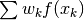where the nodes list contains the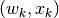pairs.

summation() will supply the list results of values computed by sum_next() at previous degrees, in case the quadrature rule is able to reuse them.

summation(f, points, prec, epsilon, max_degree, verbose=False)

Main integration function. Computes the 1D integral over the interval specified by points. For each subinterval, performs quadrature of degree from 1 up to max_degree until estimate_error() signals convergence.

summation() transforms each subintegration to the standard interval and then calls sum_next().

transform_nodes(nodes, a, b, verbose=False)

Rescale standardized nodes (for) to a general interval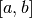. For a finite interval, a simple linear change of variables is used. Otherwise, the following transformations are used: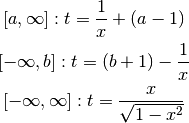### Tanh-sinh rule¶

This class implements “tanh-sinh” or “doubly exponential” quadrature. This quadrature rule is based on the Euler-Maclaurin integral formula. By performing a change of variables involving nested exponentials / hyperbolic functions (hence the name), the derivatives at the endpoints vanish rapidly. Since the error term in the Euler-Maclaurin formula depends on the derivatives at the endpoints, a simple step sum becomes extremely accurate. In practice, this means that doubling the number of evaluation points roughly doubles the number of accurate digits.

Comparison to Gauss-Legendre:
• Initial computation of nodes is usually faster
• Handles endpoint singularities better
• Handles infinite integration intervals better
• Is slower for smooth integrands once nodes have been computed

The implementation of the tanh-sinh algorithm is based on the description given in Borwein, Bailey & Girgensohn, “Experimentation in Mathematics - Computational Paths to Discovery”, A K Peters, 2003, pages 312-313. In the present implementation, a few improvements have been made:

• A more efficient scheme is used to compute nodes (exploiting recurrence for the exponential function)
• The nodes are computed successively instead of all at once

Various documents describing the algorithm are available online, e.g.:

calc_nodes(degree, prec, verbose=False)

The abscissas and weights for tanh-sinh quadrature of degreeare given by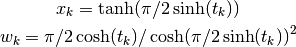where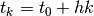for a step length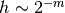. The list of nodes is actually infinite, but the weights die off so rapidly that only a few are needed.

sum_next(f, nodes, degree, prec, previous, verbose=False)

Step sum for tanh-sinh quadrature of degree. We exploit the fact that half of the abscissas at degreeare precisely the abscissas from degree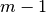. Thus reusing the result from the previous level allows a 2x speedup.

### Gauss-Legendre rule¶

This class implements Gauss-Legendre quadrature, which is exceptionally efficient for polynomials and polynomial-like (i.e. very smooth) integrands.

The abscissas and weights are given by roots and values of Legendre polynomials, which are the orthogonal polynomials onwith respect to the unit weight (see legendre()).

In this implementation, we take the “degree”of the quadrature to denote a Gauss-Legendre rule of degree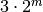(following Borwein, Bailey & Girgensohn). This way we get quadratic, rather than linear, convergence as the degree is incremented.

• Is faster for smooth integrands once nodes have been computed
• Initial computation of nodes is usually slower
• Handles endpoint singularities worse
• Handles infinite integration intervals worse
calc_nodes(degree, prec, verbose=False)

Calculates the abscissas and weights for Gauss-Legendre quadrature of degree of given degree (actually).